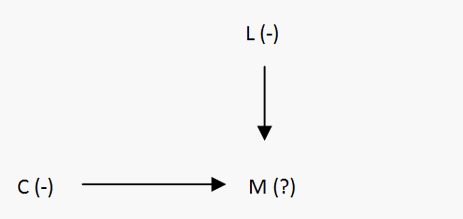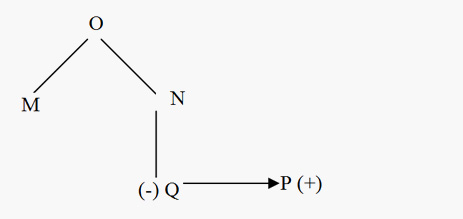# Blood Relations : Solved Examples

Example 1: Pointing to a photograph Rajan said, “He is the father of my mother’s only daughter”. How is Rajan related to the man in the photograph?
Sol: My mother’s only daughter means my sister. The Father of my sister is my father.
Therefore, that man in the photograph is Rajan's Father.
Example 2: Introducing Anil, Amit said, “He is the only son of my father’s father. How is Anil related to Amit?
Sol: My Father’s father means My Grandfather. Only son of my grandfather is my father. So, Anil is the father of Amit.
Example 3: Pointing to Kiran, Aarti said, “Her sister only brother is my Nephew. How is Aarti related to Kiran?
Sol: Here in this statement,
Kiran’s sister only brother = Kiran’s brother.
Kiran’s Brother is Aarti’s nephew. So that, Aarti is the aunt of Kiran.
Note: If we are not given any information, we will assume all the relations from the paternal perspective.
Vertical relations are used to represent the relations between father son, mother son, etc. whereas if the relationship is between the same generations then the horizontal representation is followed. We use a single arrow between the relations to mark a relation between brother and sister and similarly double arrows to represent the relationship between husband and wife.The positive sign is used to represent the males and the negative sign is used to represent females.
Example 4: C is sister of M.L is mother of M. How C is related to L?
Sol:can be clearly seen, that C is the daughter of L.
Example 5:A & B are brother & sister. M & N are brothers. A is daughter of M. How is B related to N?
Sol: It has been inferred that B is the son of M. N is brother of M. B is nephew of N.
Example 6: Mamta & Manik is a married couple. Rohit is Father of Manik .Rita is sister of Manik’s Mother’s only son. How is Mamta related to Rita?
Sol:
Manik’s Mother’s only son is Manik. Rita is sister of Manik. Mamta is wife of Manik. So Mamta is sister –in-law of Rita.
Example 7: M is brother of N, O is the father of M, P is a brother of Q, and Q is a daughter of N. Who is the uncle of P?
Sol:It can be seen from the diagram that the uncle of P is M.
Note:
In coded relation questions, the relationships are represented by specific symbols or codes like +, -, ×, ÷, *, µ, £, O etc. Then, we have to analyze some given codes & find the relation between the various persons or establish a relationship in the form of code.
Example 8: If ‘I \$ J’ means ‘I is the father of J’, ‘I *J’ means ‘I is the mother of J’, ‘I @ J’ means ‘I is the wife of J’, then which of the following means ‘K is the grandmother of L’?
1. K * T \$ L @ R
2. K * T \$ R @ L
3. K * R \$ T @ L
4. M * R @ N
5. None of these
Sol: ‘K is the grandmother of L’ means ‘K is the mother of  L's father (in this case it is T)  i.e. K * T \$ L. So, the answer is (1)
Example 9: If ‘P + Q’ means ‘P is the father of Q’, ‘P × Q’ means ‘P is the brother of Q’; ‘P –Q’ means ‘P is the mother of Q’, then which of the following is definitely true about C – A + B?
1. B is the son of A
2. A is the son of C
3. B is the father of A
4. C is the mother of B
5. None of these
Sol: C – A + B means C is the mother of A, who is the father of B. This clearly implies that A is male and hence the son of C. Hence, the answer is 2.
Example 10: If B × Q means B is the daughter of Q; B + Q means B is the father of Q; B ÷ Q means B is the mother of Q and B – Q means B is the brother of Q, then in the expression A ÷ B + C – E × F, how is A related to F?
1. Mother
2. Aunt
3. Daughter-in-law
4. Sister-in-law
5. None of these
Sol: A ÷ B + C – E × F means A is the mother of B, who is the father of C, who is the brother of E, who is the daughter of F. Thus, C and E are the children of B and F. Since B is the father, so F is the mother of C and E i.e. F is the wife of B. A is the mother of F’s husband i.e. A is the mother-in-law of F. Hence answer is 5th option.